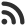Feed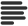Articles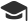Tutorials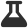Lab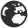CompaniesLeaderboard
 DG Student at BIT Mesra Aug. 18, 2020, 10:04 a.m. ⋅ 2229 views

# CapGemini Pseudo Code Questions with Answer and Explanation (Set 2)

This is set 2 of CapGemini Pseudo Code Questions.

Set 1 can be found from here: https://www.hackersfriend.com/articles/capgemini-pseudo-code-questions-with-answer-and-explanation-set-1

Set 3 can be found from here: https://www.hackersfriend.com/articles/capgemini-pseudo-code-questions-with-answer-and-explanation-set-3

1. What will be the output of following code :

``````#include<stdio.h>
int main(){
int i = 16;
i =! i > 15;
printf(“i = %d”,i);
return 0;
}``````

A. i = -1
B. i = 0
C. i = 1
D. Error : Undefined operation

Ans. B

Explanation : Consider the statement i =! i > 15 as :

i = negation of ( Is i > 15 )

i = negation of ( 1 ) // 1 denotes True

So, i becomes 0.

2. What will be the output of following code :

``````#include<stdio.h>
int main()
{
int x = {0, 1, 2, 3, 4, 5, 6, 7, 8, 9};
printf(“%d”,sizeof(x));
return 0;
}``````

A. 40
B. 10
C. 20
D. Error

Ans. A

Explanation : Above is the array of 10 integers and size of each integer is 4 bytes. So, 4 * 10 = 40.

3. What will be the output of following code :

``````#include<stdio.h>
int main()
{
int x = 2;
(x & 1) ? printf(“true”) : printf(“false”);
return 0;
}``````

A. true
B. false
C. 0
D. Error

Ans. B

Explanation : Above code is easy if you know the working of ternary operators. 2 & 1 is 0, so “false” is the answer.

4. What will be the output of following code :

``````#include<stdio.h>
int main()
{
int a = 4, b = 2;
printf(“a^b = %d”, a^b);
return 0;
}``````

A. 4
B. 1
C. 0
D. 6

Ans. D

Explanation : Above code is just for performing the xor operation between two variables.

5. What will be the output of following code :

``````#include<stdio.h>
int main()
{
int a = 4, b = 2;
printf(“a|b = %d\n”, a|b);
return 0;
}``````

A. 4
B. 1
C. 0
D. 6

Ans. D

Explanation : Above code is just for performing the Logical OR operation between two variables.

6. What will be the output of following code :

``````#include<stdio.h>
int main()
{
int a = NULL – true;
printf(“%d”,a);
return 0;
}``````

A. -1
B. Garbage value
C. 0
D. Error

Ans. -1

Explanation : In programming terminology, NULL denotes 0 and true denotes 1, So 0-1=> -1.

7. What will be the output of following code :

``````#include<stdio.h>
int x = 0;
int main(){
if(x == x)
printf(“if”);
else
printf(“else”);
return 0;
}``````

A. Two same variables can not be compared
B. ifelse
C. else
D. if

Ans D

Explanation : Above code involves simple comparison

8. What will be the output of following code :

``````#include<stdio.h>
#define FALSE -1
#define NULL 0
#define TRUE 1

int main(){
if(NULL)
printf(“NULL”);
else if(FALSE)
printf(“TRUE”);
else
printf(“FALSE”);
return 0;
}

``````

A. TRUE
B. FALSE
C. NULL
D. Error

Ans. A

9. What will be the output of following code :

``````#include<stdio.h>
int main(){
int i;
if(true)
printf(“work”);
else
printf(“not work”);
return 0;
}``````

A. work
B. not work
C. compiler error
D. runtime error

Ans. A

Explanation : If condition satisfies, hence work will be printed.

10. What will be the output of following code :

``````#include<stdio.h>
int main()
{
if(printf(“0”))
printf(“inside if block”);
else
printf(“inside else block”);
return 0;
}``````

A. inside else block
B. 0
C. 0inside if block
D. Error – If can not have print statement

Ans. C

Explanation : C is the correct answer as, firstly, ‘0’ will be printed and then since the condition gets fulfilled, another message ‘inside if block’ will be printed as well.

11. What will be the output of following code :

``````#include<stdio.h>
int main(){
int i = 5, j = 4;
if(!printf(“”))
printf(“%d %d”, i, j);
else
printf(“%d %d”, i++, ++j);
return 0;
}``````

A. 5 5
B. 5 4
C. 5 6
D. 6 6

Ans. B

Explanation : 5 4 will be the output as the statements inside if block will be executed. This is because :

if(printf(“”)) is false(as it is not printing any mess.) and

if( ! printf(“”)) is true

However, if there was any text written inside “” in printf(“”), then if(printf(“”)) would have become true.

12. What will be the output of following code :

``````#include<stdio.h>
int main()
{
int i = 25;
if(i == 25);
i = 50;
if(i == 25)
i = i + 1;
else
i = i + 1;
printf(“%d”, i);
return 0;
}``````

A. 51
B. 25
C. 50
D. None of these

Ans. A

Explanation : 51 is the correct answer as the first if-condition is true. So, i becomes 50. Again the second if condition is false, so corresponding else to that if statement will be executed, so i = 50 + 1 .

Events

Nov. 28, 2018, 5:30 p.m.

Python from zero to hero

place Delhi

Aug. 13, 2018, 5:30 p.m.

Python from zero to hero

place Bangalore ( HackersFriend office BTM Layout)